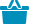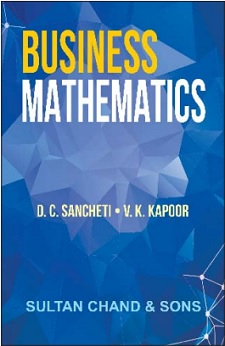Cart#### Quick Overview

Authored By

Publisher: Sultan Chand & Sons

Publishing Year: 2011

Size (mm): 220.00 x 140.00

ISBN: 81-8054-538-2

Page nos.: xvi + 1,200

MRP: 595.00

Subject: Mathematical Sciences

₹595.00

The book is designed for the students of CA, ICMA and B.Com. A complete section on linear programming has been added. Moreover a new feature is addition of application to commerce and economics and probability.

Salient Features

• A number of typical problems have been added as solved examples in each chapter. These will enable the reader to have a better and thoughtful understanding of the basic concepts of the theory and its various applications

• A conscious effort has been made to make the presentation of all topics discussed very simple and to develop the subject-matter in such step-by-step manner that the book can serve as self-study text for the students

• The book has 989 illustrations, 112 diagrams and 1,149 exercises with answers for practice.

• Logical Statements and Truth Tables
• Theory of Sets
• Boolean Algebra
• Real Number Systems
• Groups, Ring and Field
• Indices and Surds
• Logarithms
• Equations : Linear Quadratic, Cubic and Higher Order
• Permutations and Combinations
• Binomial Theorem
• Mathematical Induction, Sequence and Series
• Arithmetic and Geometric Progressions
• Convergence and Divergence of Series
• Circular Functions and Trigonometry
• Coordinate Geometry
• Functions, Limits and Continuity
• Differential Calculus
• Integral Calculus
• Vector Algebra
• Matrix Algebra. Applications to Business and Economics
• Linear  Programming
• Probability
• Tables
• Index

ISBN13: 978-81-8054-538-2

Weight: 1150.00

Edition: 11th Revised Edition

Language: English

Title Code: 552

#### Author

Authored By : Sancheti D.C., Kapoor V.K.